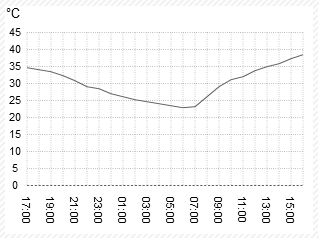## Don’t use the formula by Chow, Shao, Wang! [Power / Sample Size]

Hi David,

❝ […] on page 260 there is also another mistake, since z0.10 is 1.28 and not 0.84, so according to the reduced formula, 28 subjects per sequence would be necessary and not the reported 21.

Yep, I noticed that as well.BTW, z0.05 is 1.64 and not 1.96. In all its “beauty”:

 delta    <- log(1.25) epsilon  <- 0.05 sigma1.1 <- 0.40 z.05     <- qnorm(0.05, lower.tail=FALSE) z.10     <- qnorm(0.10, lower.tail=FALSE) (z.05+z.10)^2*sigma1.1^2/(2*(delta-epsilon)^2)  22.85316

Of course, PowerTOST contains the large sample approximation based on z as well (by an internal and hence, undocumented) function.

library(PowerTOST) CV <- seq(0.15, 0.8, 0.025) n  <- data.frame(CV=CV, exact=rep(NA, length(CV)),                  nc.t=rep(NA, length(CV)),                  shifted.t=rep(NA, length(CV)),                  normal=rep(NA, length(CV)),                  row.names=NULL) for (j in seq_along(CV)) {   # exact method (Owen's Q)   n[j, 2] <- sampleN.TOST(CV=CV[j], theta0=0.95, theta1=0.8,                           theta2=1.25, targetpower=0.8,                           method="exact", print=FALSE)[["Sample size"]]   # approximation by the noncentral t-distribution   n[j, 3] <- sampleN.TOST(CV=CV[j], theta0=0.95, theta1=0.8,                           theta2=1.25, targetpower=0.8,                           method="nct", print=FALSE)[["Sample size"]]   # approximation by the shifted central t-distribution   n[j, 4] <- sampleN.TOST(CV=CV[j], theta0=0.95, theta1=0.8,                           theta2=1.25, targetpower=0.8,                           method="shifted",                           print=FALSE)[["Sample size"]]   # (large sample) approximation by the normal distribution   n[j, 5] <- PowerTOST:::.sampleN0(se=CV2se(CV[j]), diffm=log(0.95),                                    ltheta1=log(0.8), ltheta2=log(1.25),                                    targetpower=0.8) } print(n, row.names=FALSE)

Gives:

    CV exact nc.t shifted.t normal  0.150    12   12        12     10  0.175    16   16        16     12  0.200    20   20        20     16  0.225    24   24        24     20  0.250    28   28        28     26  0.275    34   34        34     30  0.300    40   40        40     36  0.325    46   46        46     42  0.350    52   52        52     48  0.375    58   58        60     56  0.400    66   66        66     62  0.425    74   74        74     70  0.450    82   82        82     78  0.475    90   90        90     86  0.500    98   98        98     94  0.525   106  106       108    102  0.550   116  116       116    110  0.575   126  126       126    120  0.600   134  134       134    128  0.625   144  144       144    138  0.650   154  154       154    148  0.675   164  164       164    158  0.700   174  174       174    166  0.725   184  184       184    176  0.750   194  194       194    186  0.775   204  204       204    196  0.800   214  214       216    208The approximation by the noncentral t-distribution does an excellent job. That’s why we’ve set it as the default in package Power2Stage for speed reasons (~40 times faster than Owen’s Q).
The shifted central t is also good. Only in a few cases higher sample sizes. Conservative, no worries.
The large sample size approximation sucks. Always smaller sample sizes than with the other methods, power compromised – unless one dares to submit a study evaluated by z.Quoting from a conversation with an eminent regulator of the Iberian Peninsula:

“Frankly between z and t methods the difference is ridiculous when variability is not large and later a few subjects is added to compensate drop-outs. I do not see any problem in using z-method. I use it because it is very straight­forward in Excel and there is no need to have special software.”

Well, cough…

Brain-dead. 38.9 ℃ and rising, no AC in my office…Dif-tor heh smusma 🖖🏼 Довге життя Україна!Helmut SchützThe quality of responses received is directly proportional to the quality of the question asked. 🚮
Science QuotesIng. Helmut Schütz